# Swift Algorithm Club: Swift Breadth First Search

Learn how to implement a Swift breadth first search algorithm, in this step by step tutorial with diagrams and a downloadable example Playground.

The Swift Algorithm Club is an open source project to implement popular algorithms and data structures in Swift.

Every month, the SAC team will feature a cool data structure or algorithm from the club in a tutorial on this site. If your want to learn more about algorithms and data structures, follow along with us!

In this tutorial, you’ll walk through a classic of search and pathfinding algorithms, the breadth first search.

This algorithm was first implemented by Chris Pilcher, and is now refactored for tutorial format.

This tutorial assumes you have read our Swift Graphs with Adjacency Lists and Swift Queue tutorials, or have equivalent knowledge.

Note: New to the Swift Algorithm Club? Check out our getting started post first.

## Getting Started

In our tutorial on Swift Graphs with Adjacency Lists, we presented the idea of a graph as a way of expressing objects and the relationships between them. In a graph, each object is represented as a vertex, and each relationship is represented as an edge.

For example, a maze could be represented by a graph. Every junction in the maze can be represented by a vertex, and every passageway between junctions could be represented by an edge.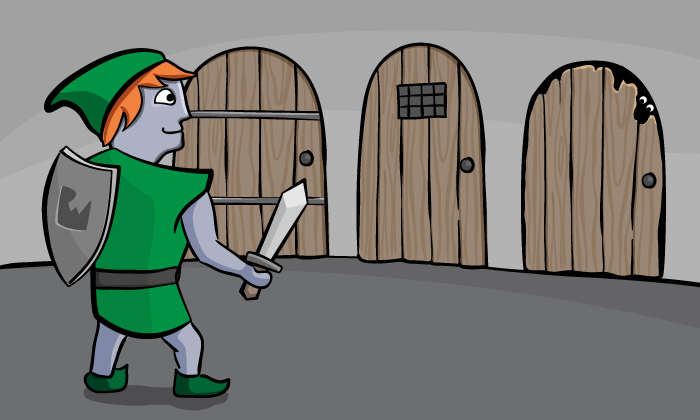Breadth first search was discovered in the 1950’s by E. F. Moore, as an algorithm not just for finding a path through a maze, but for finding the shortest path through that maze. The idea behind breadth first search is simple:

1. Explore every single location within a set number of moves of the origin.
2. Then, incrementally increase that number until the destination is found.

Let’s take a look at an example.

### An Example

Assume you are at the entrance to a maze.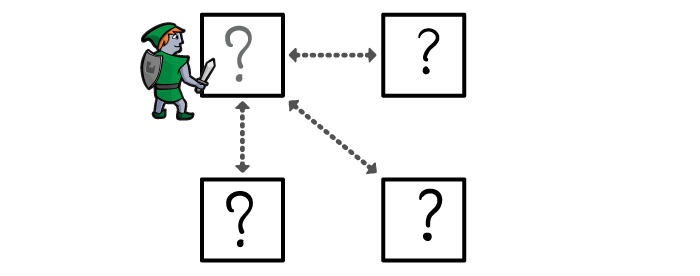The breadth first search algorithm works as follows:

1. Search your current location. If this is the destination, stop searching.
2.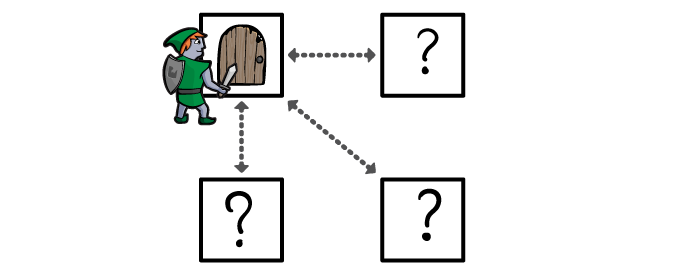3. Search the neighbors of your location. If any of them are the destination, stop searching.
4.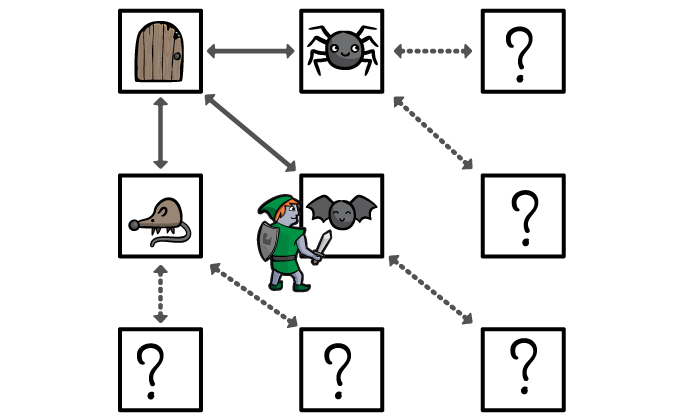5. Search all the neighbors of those locations. If any of them are the destination, stop searching.
6.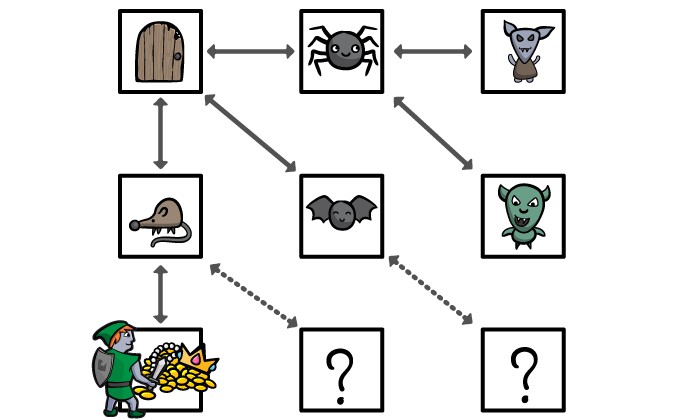7. Eventually, if there is a route to the destination, you will find it, and always in the fewest number of moves from the origin. If you ever run out of locations to search, you know that the destination cannot be reached.

Note: As far as the Breadth First Search algorithm is concerned, the shortest route means the fewest number of moves, from one location to the next.

In our maze example, the Breadth First Search algorithm treats all the passageways between rooms in the maze as if they were the same length, even though this might not be true. Think of the shortest route as being the shortest list of directions through the maze, rather than the shortest distance

We’ll explore path-finding algorithms for the shortest distance in future tutorials.

Let’s see what the breadth first search algorithm looks like in Swift.

Start by downloading the starter Playground for this tutorial, which has the data structures for a Swift adjacency list and Swift Queue included.

Note: If you’re curious how the Swift adjacency list and Swift Queue data structures work, you can see the code with View\Navigators\Show Project Navigator. You can also learn how to build these step by step in our Swift Graphs with Adjacency Lists and Swift Queue tutorials.

To recap, we defined a Graphable protocol which all graph data structures could conform to. We’re going to be extending that protocol, so we can add the breadth first search to all graphable types.

Here’s what the Graphable protocol looks like at the moment:

```public protocol Graphable {
associatedtype Element: Hashable
var description: CustomStringConvertible { get }

func createVertex(data: Element) -> Vertex<Element>
func add(_ type: EdgeType, from source: Vertex<Element>, to destination: Vertex<Element>, weight: Double?)
func weight(from source: Vertex<Element>, to destination: Vertex<Element>) -> Double?
func edges(from source: Vertex<Element>) -> [Edge<Element>]?
}
```

At the top of your playground (right after `import XCPlayground`), let’s start by creating our extension:

```extension Graphable {
public func breadthFirstSearch(from source: Vertex<Element>, to destination: Vertex<Element>)
-> [Edge<Element>]? {

}
}
```

Let’s review this function signature:

• You’ve just declared a function which takes two vertices – the source, our starting point, and the destination, our goal – and returns a route in edges which will take you from the source to the destination.
• If the route exists, you expect it to be sorted! The first edge in the route will start from the source vertex, and the last edge in the route will finish at the destination vertex. For every pair of adjacent edges in the route, the destination of the first edge will be the same vertex as the source of the second edge.
• If the source is the destination, the route will be an empty array.
• If the route doesn’t exist, the function should return nil.

Breadth first search relies on visiting the vertices in the correct order. The first vertex to visit will always be the source. After that we’ll explore the source vertex’s neighbors, then their neighbors and so on. Every time we visit a vertex, we add its neighbors to the back of the queue.

We’ve encountered Queues before, so here’s an excellent opportunity to use one!

```public func breadthFirstSearch(from source: Vertex<Element>, to destination: Vertex<Element>)
-> [Edge<Element>]? {

var queue = Queue<Vertex<Element>>()
queue.enqueue(source) // 1

while let visitedVertex = queue.dequeue() { // 2
if visitedVertex == destination { // 3
return []
}
// TODO...
}
return nil // 4

}
```

Let’s review this section by section:

1. This creates a queue for vertices, and enqueues the source vertex.
2. This dequeues a vertex from the queue (as long as the queue isn’t empty) and calls it the visited vertex.

In the first iteration, the visited vertex will be the source vertex and the queue will immediately be empty. However, if visiting the source vertex adds more vertices to the loop, the search will continue.

3. This checks whether the visited vertex is the destination. If it is, the search ends immediately. For now you return an empty list, which is the same as saying the destination was found. Later you’ll compose a more detailed route.
4. If the queue runs out of vertices, you return nil. This means the destination wasn’t found, and no route to it is possible.

We now need to enqueue the visited vertex’s neighbors. Replace the TODO with the following code:

```let neighbourEdges = edges(from: visitedVertex) ?? [] // 1
for edge in neighbourEdges {
queue.enqueue(edge.destination)
} // 2
```

Let’s review this section by section:

1. This uses the Graphable protocol’s `edges(from:)` method to get the array of edges from the visited vertex. Remember that the `edges(from:)` function returns an optional array of edges. This means if the array is empty, or nil, then there are no edges beginning with that vertex.

Because, for the purposes of our search, the empty list and nil mean the same thing – no neighbors to add to the queue – we’ll nil-coalesce the optional array with the empty list to remove the optional.

2. You can now safely use a for-loop with the list of edges, to enqueue each edge’s destination vertex.

We’re not quite done here yet. There’s a subtle danger in this search algorithm! What problem would you run into if you ran the search algorithm on this example? Ignore the fact that the treasure room isn’t connected to the graph.

Work out what happens every time we visit a vertex with pen and paper, if that helps.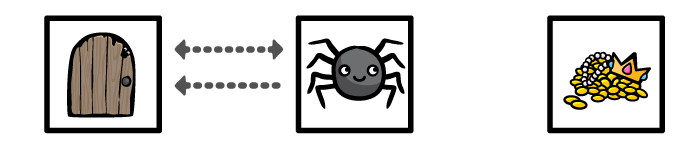[spoiler title=”What problem would you run into if you ran the search algorithm on this example?”]

1. The breadth first search function creates a queue, and enqueues the entrance room.
2. The first time we go through the while loop, we dequeue the entrance room and visit it. The entrance room doesn’t have the treasure, so we explore the entrance room’s neighbors. The entrance room has one neighbor, the spider room, so we enqueue that.
3. The second time we go through the while loop, we dequeue the spider room and visit it. The spider room doesn’t have the treasure, so we explore the spider room’s neighbors. The spider room has one neighbor, the entrance room, so we enqueue that.
4. The third time we go through the while loop, we dequeue the entrance room…

The problem is: we’ve been here before!

To fix this problem, We need to remember the vertices we visit so we don’t visit them more than once.
[/spoiler]

There are several ways to do this. Update your code to this:

```public func breadthFirstSearch(from source: Vertex<Element>, to destination: Vertex<Element>) -> [Edge<Element>]? {
var queue = Queue<Vertex<Element>>()
queue.enqueue(source)
var enqueuedVertices = Set<Vertex<Element>>() // 1

while let visitedVertex = queue.dequeue() {
if visitedVertex == destination {
return []
}
let neighbourEdges = edges(from: visitedVertex) ?? []
for edge in neighbourEdges {
if !enqueuedVertices.contains(edge.destination) { // 2
enqueuedVertices.insert(visitedVertex) // 3
queue.enqueue(edge.destination)
}
}
}
return nil
}
```

Let’s review what’s changed:

1. This creates a set of vertices, to represent the list of vertices you’ve encountered so far. Remember that the Vertex type is `Hashable`, so we don’t need to do any more work than this to make a set of vertices.
2. Whenever you examine a neighboring vertex, you first check to see if you’ve encountered it so far.
3. If you haven’t encountered it before, you add it to both queues: the list of “vertices to process” (`queue`) and the list of “vertices encountered” (`enqueuedVertices`).

This means the search is considerably safer. You now can’t visit more vertices than are in the graph to begin with, so the search must eventually terminate.

### Finding the Way Back

You’re almost done!

At this point, you know that if the destination can’t be found, you’ll return nil. But if you do find the destination, you need to find your way back. Unfortunately, every room you’ve visited, you’ve also dequeued, leaving no record of how you found the destination!

To keep a record of your exploration, you’re going to replace your set of explored vertices with a dictionary, containing all your explored vertices and how you got there. Think of it as exploring a maze, and leaving a chalk arrow pointing towards all the rooms you explored – and when you come back to a room, following the directions the arrows are pointing from, to get back to the entrance.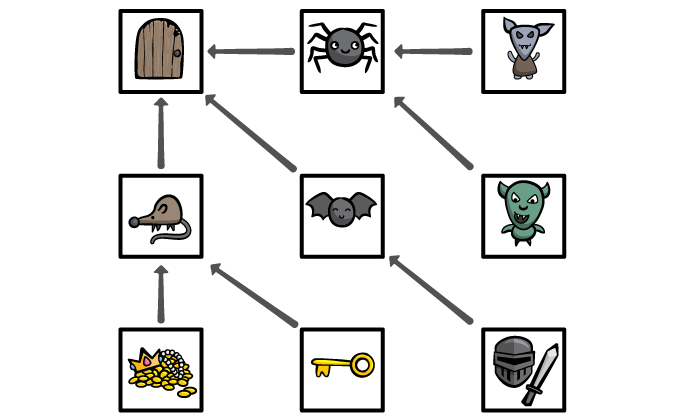If we kept track of all the arrows we drew, for any room we’ve visited, we can just look up the edge we took to get to it. That edge will lead back to a room we visited earlier, and we can look up the edge we took to get there as well, and so on back to the beginning.

Let’s try this out, starting by creating the following Visit enum type. You’ll have to create this outside the Graphable extension, because Swift 3 doesn’t allow nested generic types.

```enum Visit<Element: Hashable> {
case source
case edge(Edge<Element>)
}
```

We’re being clear and Swifty here. In our look-up table, every item in the first column was a Vertex, but not every item in the second column is an Edge; one Vertex will always be the source vertex. If not, something has gone badly wrong and we’ll never get out of the graph!

Next modify your method as follows:

```public func breadthFirstSearch(from source: Vertex<Element>, to destination: Vertex<Element>) -> [Edge<Element>]? {
var queue = Queue<Vertex<Element>>()
queue.enqueue(source)
var visits : [Vertex<Element> : Visit<Element>] = [source: .source] // 1

while let visitedVertex = queue.dequeue() {
// TODO: Replace this...
if visitedVertex == destination {
return []
}
let neighbourEdges = edges(from: visitedVertex) ?? []
for edge in neighbourEdges {
if visits[edge.destination] == nil { // 2
queue.enqueue(edge.destination)
visits[edge.destination] = .edge(edge) // 3
}
}
}
return nil
}
```

Let’s review what’s changed here:

1. This creates a Dictionary of Vertex keys and Visit values, and initializes it with the source vertex as a ‘source’ visit.
2. If the Dictionary has no entry for a vertex, then it hasn’t been enqueued yet.
3. Whenever you enqueue a vertex, you don’t just put the vertex into a set, you record the edge you took to reach it.

Finally, you can backtrack from the destination to the entrance! Update that if-statement with the TODO to this:

```if visitedVertex == destination {
var vertex = destination // 1
var route: [Edge<Element>] = [] // 2

while let visit = visits[vertex],
case .edge(let edge) = visit { // 3

route = [edge] + route
vertex = edge.source // 4

}
return route // 5
}
```

Let’s review this section by section:

1. You created a new variable, to store each vertex which is part of the route.
2. You also created a variable to store your route.
3. You created a while-loop, which will continue as long as the visits Dictionary has an entry for the vertex, and as long as that entry is an edge. If the entry is a source, then the while-loop will end.
4. You added that edge to the start of your route, and set the vertex to that edge’s source. You’re now one step closer to the beginning.
5. The while-loop has ended, so your route must now be complete.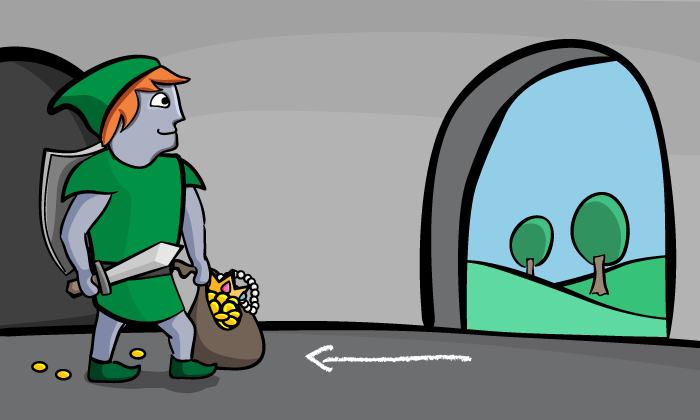That’s it! You can test this out by adding the following to the end of your playground:

```if let edges = dungeon.breadthFirstSearch(from: entranceRoom, to: treasureRoom) {
for edge in edges {
print("\(edge.source) -> \(edge.destination)")
}
}
```

You should see the following print out in your console:

```Entrance -> Rat
Rat -> Treasure
```

## Where To Go From Here?

I hope you enjoyed this tutorial on the Swift breadth first search algorithm!

You’ve extended the behavior of all Graphable data types, so you can search for a route from any vertex to any other vertex. Better still, you know it’s a route with the shortest number of steps.

Here is a playground with the above code. You can also find the original implementation and further discussion in the breadth first search section of the Swift Algorithm Club repository.

This was just one of the many algorithms in the Swift Algorithm Club repository. If you’re interested in more, check out the repo.

It’s in your best interest to know about algorithms and data structures – they’re solutions to many real world problems, and are frequently asked as interview questions. Plus it’s fun!

So stay tuned for many more tutorials from the Swift Algorithm club in the future. In the meantime, if you have any questions on implementing breadth first search in Swift, please join the forum discussion below!

Note: The Swift Algorithm Club is always looking for more contributors. If you’ve got an interesting data structure, algorithm, or even an interview question to share, don’t hesitate to contribute! To learn more about the contribution process, check out our Join the Swift Algorithm Club article.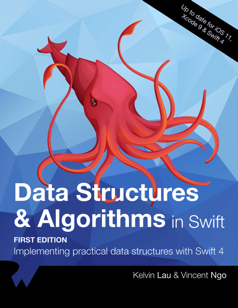If you enjoyed what you learned in this tutorial, why not check out our Data Structures and Algorithms in Swift book, available on our store?

In Data Structures and Algorithms in Swift, you’ll learn how to implement the most popular and useful data structures and when and why you should use one particular datastructure or algorithm over another. This set of basic data structures and algorithms will serve as an excellent foundation for building more complex and special-purpose constructs.

As well, the high-level expressiveness of Swift makes it an ideal choice for learning these core concepts without sacrificing performance.

• You’ll start with the fundamental structures of linked lists, queues and stacks, and see how to implement them in a highly Swift-like way.
• Move on to working with various types of trees, including general purpose trees, binary trees, AVL trees, binary search trees and tries.
• Go beyond bubble and insertion sort with better-performing algorithms, including mergesort, radix sort, heap sort and quicksort.
• Learn how to construct directed, non-directed and weighted graphs to represent many real-world models, and traverse graphs and trees efficiently with breadth-first, depth-first, Dijkstra’s and Prim’s algorithms to solve problems such as finding the shortest path or lowest cost in a network.
• And much, much more!

By the end of this book, you’ll have hands-on experience solving common issues with data structures and algorithms — and you’ll be well on your way to developing your own efficient and useful implementations.